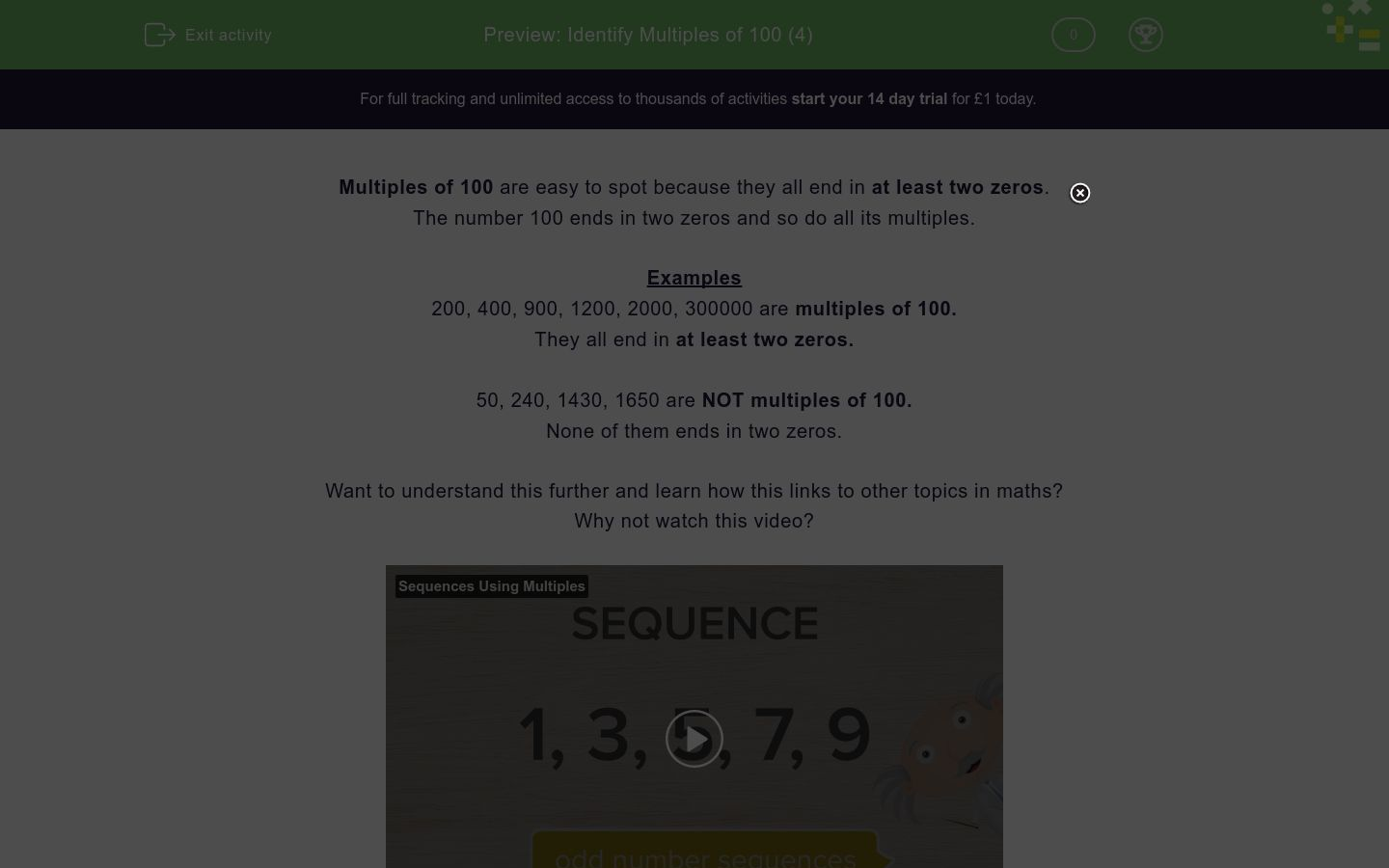# Identify Multiples of 100 (4)Key stage:  KS 2

Curriculum topic:   Number: Number and Place Value

Curriculum subtopic:   Count in Multiples (4, 8, 50 and 100)

Difficulty level:\$appendString = '&new=1'; \$_SESSION['guest_aId'] = rand(); \$urlToRedirect = FRAMEWORK_PATH . 'preview/worksheet/introduction?aId=' . \$_SESSION['guest_aId'] . '&wId=' . \$wsData['id'] . '&usertype=guest' . \$appendString; ?>

### QUESTION 1 of 10

Multiples of 100 are easy to spot because they all end in at least two zeros.

The number 100 ends in two zeros and so do all its multiples.

Examples

200, 400, 900, 1200, 2000, 300000 are multiples of 100.

They all end in at least two zeros.

50, 240, 1430, 1650 are NOT multiples of 100.

None of them ends in two zeros.

Want to understand this further and learn how this links to other topics in maths?
Why not watch this video?

Look at this list of numbers.

31, 72, 200, 209, 910

Which number is a multiple of 100?

Look at this list of numbers.

300, 720, 20, 309, 460, 991

Which number is a multiple of 100?

Look at this list of numbers.

30, 780, 50, 709, 400, 990, 480

Which number is a multiple of 100?

Look at this list of numbers.

50, 750, 70, 705, 507, 770, 500, 1050

Which number is a multiple of 100?

Look at this list of numbers.

60, 760, 70, 706, 607, 770, 670, 7060, 600

Which number is a multiple of 100?

Look at this list of numbers.

30, 380, 80, 308, 800, 880, 830, 3080, 330

Which number is a multiple of 100?

Look at this list of numbers.

30, 390, 90, 909, 9909, 990, 930, 9030, 300, 93

Which number is a multiple of 100?

Look at this list of numbers.

20, 1000, 120, 1020, 10, 12

Which number is a multiple of 100?

Look at this list of numbers.

20, 990, 920, 9020, 2000, 92

Which number is a multiple of 100?

Look at this list of numbers.

34, 40, 430, 440, 4000, 404, 4430, 334, 43

Which number is a multiple of 100?

• Question 1

Look at this list of numbers.

31, 72, 200, 209, 910

Which number is a multiple of 100?

200
EDDIE SAYS
200 is the only number with at least two zeros.
• Question 2

Look at this list of numbers.

300, 720, 20, 309, 460, 991

Which number is a multiple of 100?

300
EDDIE SAYS
300 is the only number with at least two zeros.
• Question 3

Look at this list of numbers.

30, 780, 50, 709, 400, 990, 480

Which number is a multiple of 100?

400
EDDIE SAYS
400 is the only number with at least two zeros.
• Question 4

Look at this list of numbers.

50, 750, 70, 705, 507, 770, 500, 1050

Which number is a multiple of 100?

500
EDDIE SAYS
500 is the only number with at least two zeros.
• Question 5

Look at this list of numbers.

60, 760, 70, 706, 607, 770, 670, 7060, 600

Which number is a multiple of 100?

600
EDDIE SAYS
600 is the only number with at least two zeros.
• Question 6

Look at this list of numbers.

30, 380, 80, 308, 800, 880, 830, 3080, 330

Which number is a multiple of 100?

800
EDDIE SAYS
800 is the only number with at least two zeros.
• Question 7

Look at this list of numbers.

30, 390, 90, 909, 9909, 990, 930, 9030, 300, 93

Which number is a multiple of 100?

300
EDDIE SAYS
300 is the only number with at least two zeros.
• Question 8

Look at this list of numbers.

20, 1000, 120, 1020, 10, 12

Which number is a multiple of 100?

1000
EDDIE SAYS
1000 is the only number with at least two zeros.
• Question 9

Look at this list of numbers.

20, 990, 920, 9020, 2000, 92

Which number is a multiple of 100?

2000
EDDIE SAYS
2000 is the only number with at least two zeros.
• Question 10

Look at this list of numbers.

34, 40, 430, 440, 4000, 404, 4430, 334, 43

Which number is a multiple of 100?

4000
EDDIE SAYS
4000 is the only number with at least two zeros.
---- OR ----

Sign up for a £1 trial so you can track and measure your child's progress on this activity.

### What is EdPlace?

We're your National Curriculum aligned online education content provider helping each child succeed in English, maths and science from year 1 to GCSE. With an EdPlace account you’ll be able to track and measure progress, helping each child achieve their best. We build confidence and attainment by personalising each child’s learning at a level that suits them.

Get started